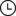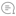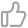# 撸了个搜索引擎系统，爽！互联网架构师 |872022-04-09 21:46000

Q技能 阿尔法突袭

W技能 冥想

E技能 无双

R技能 高原血统1.蛮王

2.无极剑圣

3.剑姬

1.索引模块

1）扫描下载到的文档，分析内容，构建出，正排索引和倒排索引。并且把索引内容保存到文件中。

2）加载制作i好的索引。并提供一些API实现查正排和查倒排这样的功能。

2.搜索模块

1）调用索引模块，实现一个搜索的完整过程。

3.web模块

1.基于词库

2.基于统计

java中能够实现分词的第三方工具也是有很多的test包里直接操作：我们使用这个测试代码直接搞。试一下这个包咋用。``import org.ansj.domain.Term;``import org.ansj.splitWord.analysis.ToAnalysis;``import java.util.List;``public class TastAnsj {``    public static void main(String[] args) {``        String str = "易大师是一个有超高机动性的刺客、战士型英雄，擅长利用快速的打击迅速击溃对手，易大师一般打野和走单人路，作为无极剑道的最后传人，易可以迅速砍出大量伤害，同时还能利用技能躲避猛烈的攻击，避开敌人的集火。";``        List<Term> terms = ToAnalysis.parse(str).getTerms();``        for (Term term : terms) {``            System.out.println(term.getName());``        }``    }``}``

``import java.io.File;``import java.util.ArrayList;``//读取刚刚文档``public class Parser {``     private static final  String INPUT_PATH="D:/test/docs/api";``      public  void run(){``          //整个Parser类的入口``          //1.根据路径，去枚举出所有的文件.（html）；``          ArrayList<File> fileList=new ArrayList<>();``          enumFile(INPUT_PATH,fileList);``          System.out.println(fileList);``          System.out.println(fileList.size());``          //2.针对上面罗列出的文件，打开文件，读取文件内容，并进行解析``          //3.把在内存中构造好的索引数据结构，保定到指定的文件中。``      }``      //第一个参数表示从哪里开始遍历 //第二个表示结果。``      private void enumFile(String inputPath,ArrayList<File>fileList){``         File rootPath=new File(inputPath);``         //listFiles 能够获取到一层目录下的文件``        File[] files= rootPath.listFiles();``         for(File f:files){``             //根据当前f的类型判断是否递归。``             //如果f是一个普通文件，就把f加入到fileList里面``             //如果不是就调用递归``             if(f.isDirectory()){``                 enumFile(f.getAbsolutePath(),fileList);``             }else {``                 fileList.add(f);``             }``         }``      }``    public static void main(String[] args) {``        //通过main方法来实现整个制作索引的过程``        Parser parser=new Parser();``        parser.run();``    }``}``

``else {``                 if(f.getAbsolutePath().endsWith(",html"))``                 fileList.add(f);``             }``

1. 打开文件，解析内容。

1.1解析Title

f.getName()是直接读取文件名字的方法。

``private  String parseTitle(File f) {``          String name= f.getName();``         return name.substring(0,f.getName().length()-5);``    }``

1.2解析Url操作

``private  String parseUrl(File f) {``      String part1="https://docs.oracle.com/javase/8/docs/api/";``      String part2=f.getAbsolutePath().substring(INPUT_PATH.length());``          return part1+part2;``    }``

1.3解析内容

``private  String parseContent(File f) throws IOException {``          //先按照一个一个字符来读取，以<>作为开关``        try(FileReader fileReader=new FileReader(f)) {``            //加上一个是否拷贝的开关.``            boolean isCopy=true;``            //还需要准备一个结果保存``            StringBuilder content=new StringBuilder();``            while (true){``                //此处的read的返回值是int，不是char``                //如果读到文件末尾，就会返回-1，这是用int的好处；``                int  ret = 0;``                try {``                    ret = fileReader.read();``                } catch (IOException e) {``                    e.printStackTrace();``                }``                if(ret==-1) {``                        break;``                    }``                    char c=(char) ret;``                    if(isCopy){``                        if(c=='<'){``                            isCopy=false;``                            continue;``                        }``                        //其他字符直接拷贝``                        if(c=='\n'||c=='\r'){``                            c=' ';``                        }``                        content.append(c);``                    }else{``                        if(c=='>'){``                            isCopy=true;``                        }``                    }``            }``            return  content.toString();``        } catch (FileNotFoundException e) {``            e.printStackTrace();``        }``        return "";``    }``

``import java.io.File;``import java.io.FileNotFoundException;``import java.io.FileReader;``import java.io.IOException;``import java.util.ArrayList;``//读取刚刚文档``public class Parser {``     private static final  String INPUT_PATH="D:/test/docs/api";``      public  void run(){``          //整个Parser类的入口``          //1.根据路径，去枚举出所有的文件.（html）；``          ArrayList<File> fileList=new ArrayList<>();``          enumFile(INPUT_PATH,fileList);``          System.out.println(fileList);``          System.out.println(fileList.size());``          //2.针对上面罗列出的文件，打开文件，读取文件内容，并进行解析``          for (File f:fileList){``              System.out.println("开始解析"+f.getAbsolutePath());``              parseHTML(f);``          }``          //3.把在内存中构造好的索引数据结构，保定到指定的文件中。``      }``    private  String parseTitle(File f) {``          String name= f.getName();``         return name.substring(0,f.getName().length()-5);``    }``    private  String parseUrl(File f) {``      String part1="https://docs.oracle.com/javase/8/docs/api/";``         String part2=f.getAbsolutePath().substring(INPUT_PATH.length());``          return part1+part2;``    }``    private  String parseContent(File f) throws IOException {``          //先按照一个一个字符来读取，以<>作为开关``        try(FileReader fileReader=new FileReader(f)) {``            //加上一个是否拷贝的开关.``            boolean isCopy=true;``            //还需要准备一个结果保存``            StringBuilder content=new StringBuilder();``            while (true){``                //此处的read的返回值是int，不是char``                //如果读到文件末尾，就会返回-1，这是用int的好处；``                int  ret = 0;``                try {``                    ret = fileReader.read();``                } catch (IOException e) {``                    e.printStackTrace();``                }``                if(ret==-1) {``                        break;``                    }``                    char c=(char) ret;``                    if(isCopy){``                        if(c=='<'){``                            isCopy=false;``                            continue;``                        }``                        //其他字符直接拷贝``                        if(c=='\n'||c=='\r'){``                            c=' ';``                        }``                        content.append(c);``                    }else{``                        if(c=='>'){``                            isCopy=true;``                        }``                    }``            }``            return  content.toString();``        } catch (FileNotFoundException e) {``            e.printStackTrace();``        }``        return "";``    }``    private void parseHTML (File f){``        //解析出标题``          String title=parseTitle(f);``        //解析出对应的url``          String url=parseUrl(f);``        //解析出对应的正文``        try {``            String content=parseContent(f);``        } catch (IOException e) {``            e.printStackTrace();``        }``    }``      //第一个参数表示从哪里开始遍历 //第二个表示结果。``      private void enumFile(String inputPath,ArrayList<File>fileList){``         File rootPath=new File(inputPath);``         //listFiles 能够获取到一层目录下的文件``        File[] files= rootPath.listFiles();``         for(File f:files){``             //根据当前f的类型判断是否递归。``             //如果f是一个普通文件，就把f加入到fileList里面``             //如果不是就调用递归``             if(f.isDirectory()){``                 enumFile(f.getAbsolutePath(),fileList);``             }else {``                 if(f.getAbsolutePath().endsWith(".html"))``                 fileList.add(f);``             }``         }``      }``    public static void main(String[] args) {``        //通过main方法来实现整个制作索引的过程``        Parser parser=new Parser();``        parser.run();``    }``}``

· END ·

1.救救大龄码农！45岁程序员在国务院网站求助总理!央媒网评来了...00

暂无评论~~
Ctrl+Enter